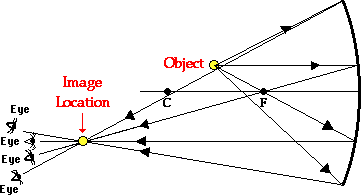Home » Posts tagged 'concave mirror'

# Tag Archives: concave mirror

## Optics MCQ – image formation by concave mirror

I am having trouble with a problem.

Q. In an experiment to determine the focal length (f) of a concave mirror by the u–v method, a student places the object pin A on the principal axis at a distance x from the pole P. The student looks at the pin and its inverted image from a
distance keeping his/her eye in line with PA. When the student shifts his/her eye towards left, the image appears to the right of the object pin. Then

(A) x < f

(B) f < x < 2f

(C) x = 2f

(D) x > 2fEXPLANATION

The image and the object will move together at 2f

The one closer to the eye will move faster

In the question, the situation occurs when the image is closer to the eye and this happens when the object is between f and 2f

## Five Sure Shot portions for SA2 (Class X CBSE)

The students appearing for SA2 of CBSE class X are requested to practice the following portions of Physics very well so that they can get excellently good marks in Science.

1. Practice all ray diagrams showing image formation by concave mirror, convex mirror, convex lens and concave lens along with characteristics of image.
2. Defects of eye, cause and rectification
3. Structure of human eye (diagram), function of each part, power of accommodation and persistence of vision.
4. Refraction through a glass slab and a glass prism, lateral displacement and angle of deviation.
5. Scattering of light, why sky is blue, Tyndal effect.
6. Also Practice numerical based on mirror formula, lens formula, refractive index.

## Questions normally not answered by Students in Viva

Here are some questions which are not properly answered by students during Viva.

1. Name the Physical quantities to be kept constant for Ohm’s law to be true.
2. State the Principle of a potentiometer. (The students say that potential drop is proportional to length but the constant quantities are not mentioned)
3. How can we increase the sensitivity of a potentiometer?
4. Define figure of merit of a galvanometer.
5. Which has more resistance – a galvanometer or a milliammeter?
6. How does an LED emit light?
7. What is the difference between an ordinary diode and an LED?
8. Define principal axis of a convex lens?
9. What happens to the focal length of a concave mirror if it is immersed in water?
10. What are the factors affecting the intrernal resistance of a cell?
11. What are the difference between primary and secondary cell?
12. Why can’t we use a dry cell for starting a car?

1. length, area of cross section,temperature
2. The potential drop across any length of a conductor of uniform cross section and composition carrying a constant current is directly proportional to the length.
3. increasing the length of potentiometer wire, decreasing the current, decreasing the potential gradient
4. Current for unit deflection
5. galvanometer
6. The energy released during recombination of electrons and holes across the junction is responsible for the release of light by LED
7. In ordinary diode the energy emitted during recombination of electrons and holes is in the invisible region of the em spectrum but in the case of LED, the energy is in the visible region.
8. Straight line joining the centres of curvature of the lens..
9. The focal length of mirror does not change by changing the medium. Their is a pure geometrical relationship between the radius of curvature and focal length and the relation does not include any term depending on refractive index.
10. the nature of electrolyte, the concentration of electrolyte, temperature, distance between electrodes, area of electrodes
11. Primary cell cannot be recharged, secondary cells can be recharged; secondary cells have less internal resistance than primary cells.
12. Due to the high internal resistance of a dry cell, it won’t be able to provide the current sufficient to start the car

## A numerical Problem from optics

When an object is kept at a distance of 60cm from a concave mirror, the magnification is 1/2. Where should the object be placed to get magnification of 1/3??

Ans:

In the first part, u=-60, m=(-)1/2 implies v=-30 cm (Since the image must be real as concave lens form virtual image of bigger size only)

we get f = –20 cm

In II case

m=-1/3

v=u/3

f=-20cm

we get u=4f=4 x (-20)=-80 cm